# Data Analytics: Python Basics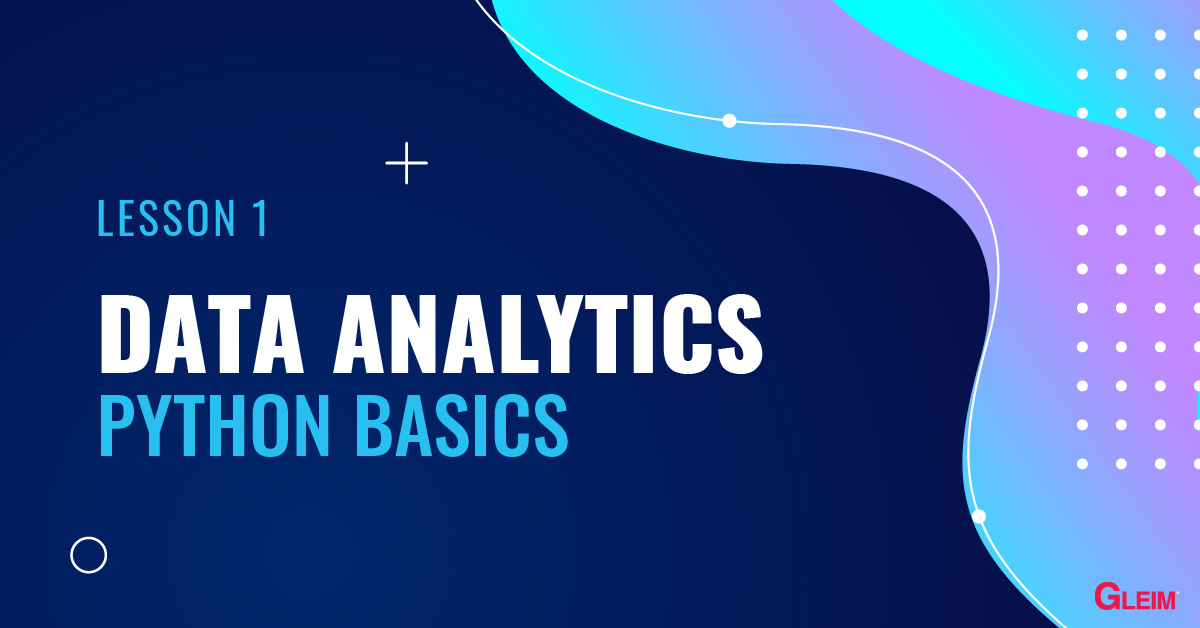Python is a relatively simple programming language with an English-like syntax. Due to its simplicity and numerous available libraries, Python is gaining increasing popularity throughout the world, especially in the field of data analytics. This series of blogs introduces the basics of Python.

## Using Python

There are three environments you can use to run Python code. We will use the notebook environment for this blog series.

The notebook environment runs the code cell-by-cell, thereby allowing us to see both the input and output at the same time. The most popular notebook environment is the Jupyter Notebook or JupyterLab, which is available for free with the Anaconda package manager.

To open a notebook environment,

1. Launch the Anaconda Navigator.
• JupyterLab has the same notebook interface as Jupyter Notebook but contains more functionality. For illustration purposes, we will use JupyterLab.
3. In the Launcher page, select Python 3 under Notebook to create a new Python notebook.

Below is an example of an empty Python notebook.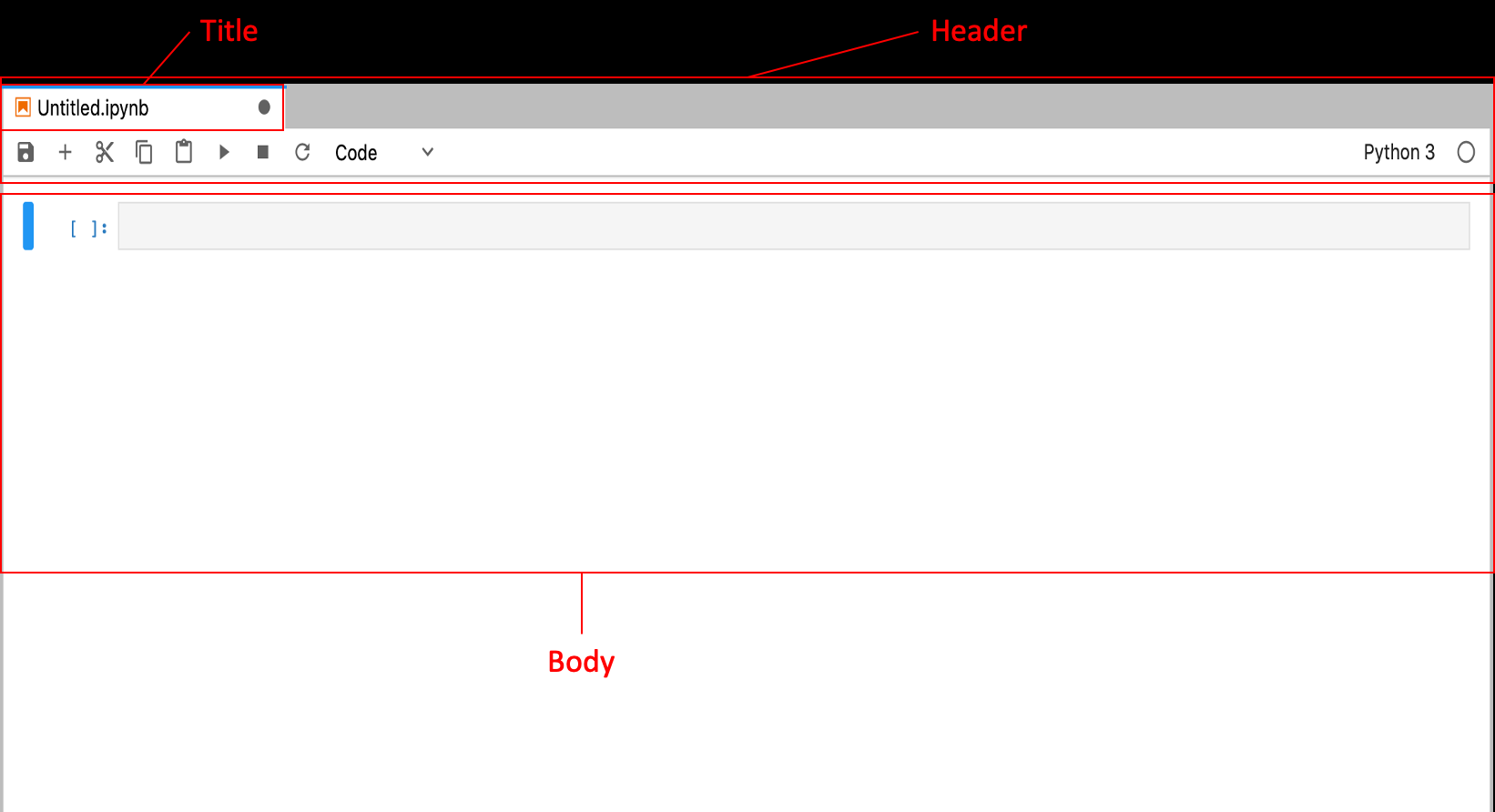Header: The Header contains the Title of the notebook and the toolbar.

Toolbar: The toolbar contains a variety of actions that control the notebook.

Title: When a new notebook is created, the notebook is named Untitled. Right-click the Title and choose Rename Notebook to rename the notebook to “Python Basics.”

Body: The body of a notebook is composed of cells. Cells are where text is entered or code is run.

Markdown cells: These cells are for the entry of markdown text and are not preceded by square brackets. Markdown cells contain text meant for human readers Opens in new window.

Code cells: These cells are for running code. A code cell is preceded by square brackets. When code is run in the code cells, the output of the code is displayed below the code cell and a number is displayed within the square bracket to indicate the order of execution of the code cells.

Shortcut Key Combination Cell Operation
In Command Mode: Cell border is gray, use esc to activate the mode
Ctrl + enter Run the cell
Shift + enter Run the cell and select the cell below
Alt + enter Run the cell and insert a cell below
A Insert a cell above
B Insert a cell below
C Copy a cell
V Paste a copied cell
D D Delete a selected cell
Y Change a cell to a code cell
M Change a cell to a markdown cell
0 0 (Zero, Zero) Restart the whole Kernel (the order of execution resets to 1)
H View all keyboard shortcuts

To run all the cells from the beginning , select Kernel > Restart Kernel and Run All Cells.

## Variables

A variable is the storage of value. In the code cells, a variable can be assigned any value and name. To assign a value to a variable, type the name of the variable, followed by = and the value.

Example:
In the code cell, type the following: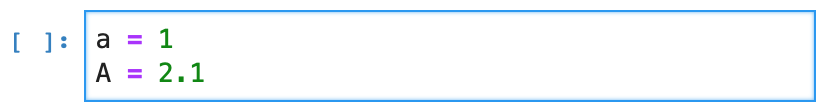Press   Shift + Enter   to run the code cell and assign the value of 1 to the variable “a” and the value of 2.1 to the variable “A.” Note that Python variables are case-sensitive; thus, the variable “a” differs from “A.”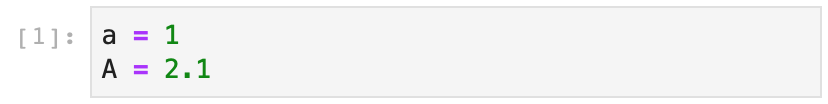To see the value assigned to or use a variable, type the name of the variable in a code cell and the output box will display its value.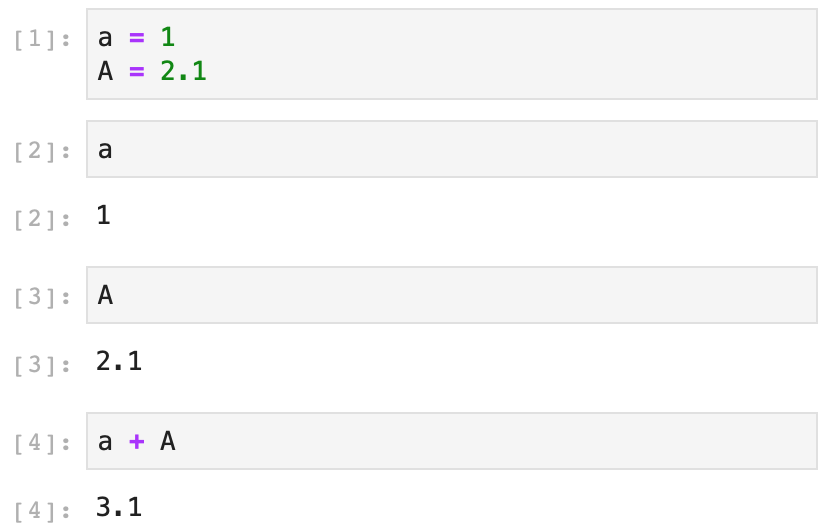The   print( )   function can also be used to display the result.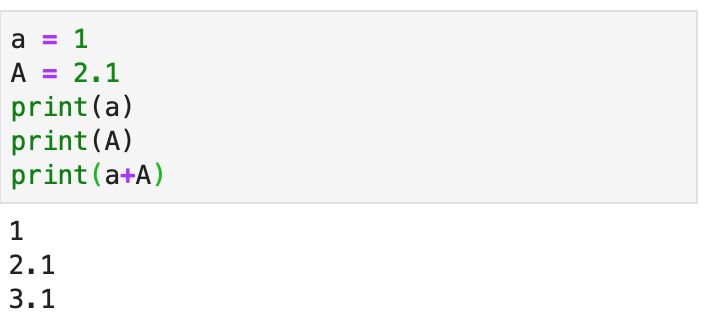## Data Types

Data types are the types of values that can be stored in variables. Below is a list of the data types:

### Numbers

Python mainly supports 2 types of numbers:
int     Integers (whole numbers), such as 1, 4, and 7 (e.g., the value assigned to the variable “a”).
float     Floating-point numbers (number with decimal digits), such as 3.14159 and 9.81 (e.g., the value assigned to the variable “A”).

### Strings

str     Strings are similar to text data and are threads of characters enclosed in quotation marks, such as “Aaron,” “text,” etc. Numbers enclosed in quotation marks are treated as strings.

Example: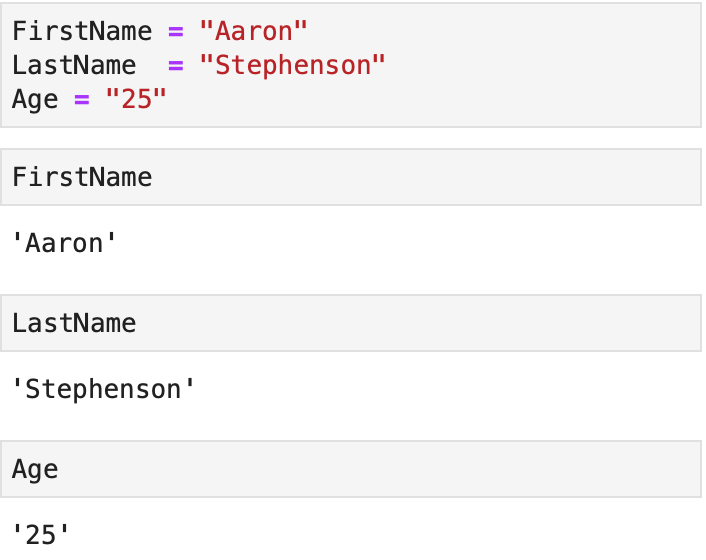Double quotation marks are preferred to single quotation marks to allow apostrophes’ in the string.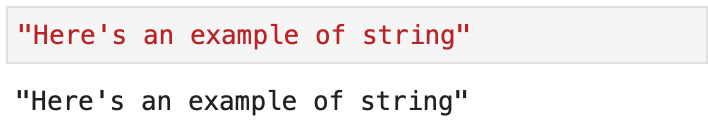Because a string is a combination of characters, each character in a string can be accessed by square brackets and an index. A space in a string is considered a character. The indexes of the characters start from number 0, with negative numbers denoting the indexes in reverse order.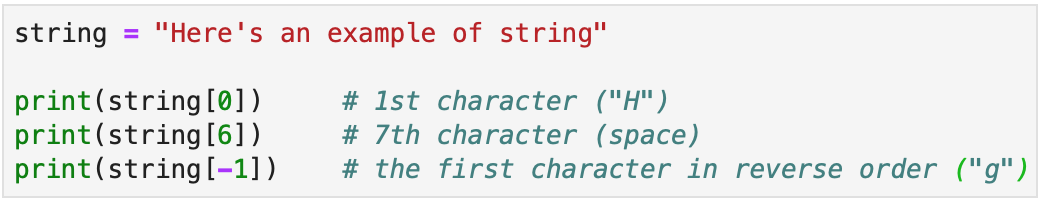The indexes can also access a slice of a string using slice notations. Below are the slice notations used in Python. Note that the stopping index is not included.

Notation Meaning
StringName[start : stop] From starting index through stop – 1
StringName[:] The whole string
StringName[start : ] From starting index to the end
StringName[ : stop] From beginning to the stopping index (exclusive)

### Boolean

bool   Boolean values take only True or False. They are the results of logical tests.
Example: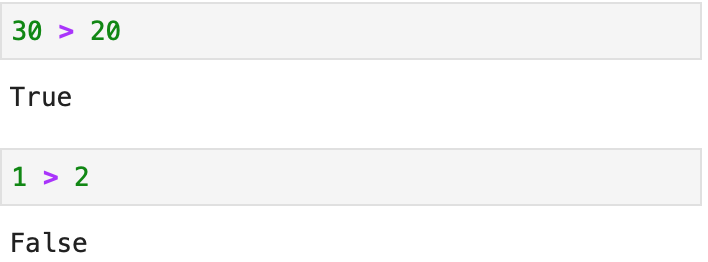## Data Sequences

### List

list     Lists are ordered lists with mutable elements. Elements of a list are put in square brackets.

Example: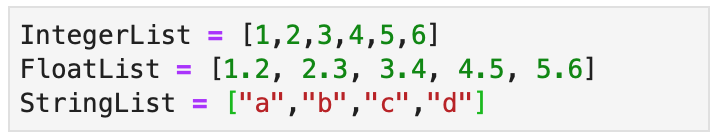Elements of a list are indexed from zero.

Notation Meaning
ListName[start : stop] From starting index through stop – 1
ListName[:] The whole string
ListName[start : ] From starting index to the end
ListName[ : stop] From beginning to the stopping index (exclusive)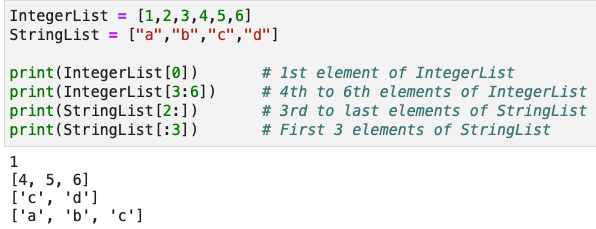Elements of a list can be of any data type.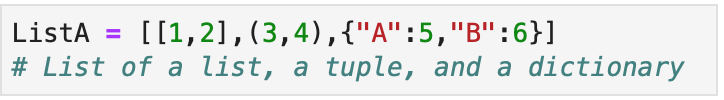To add elements to the end of a list, use the  .append( )   function.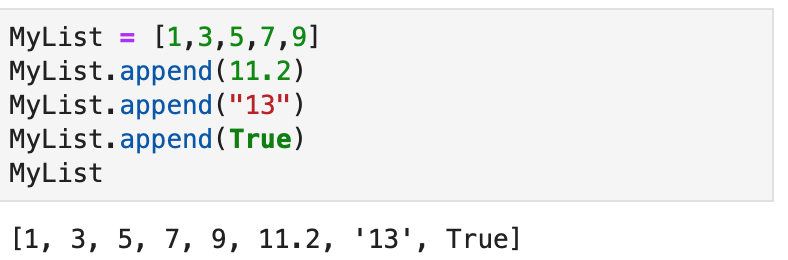To add a list to the end of another list, use the  .extend( )   function.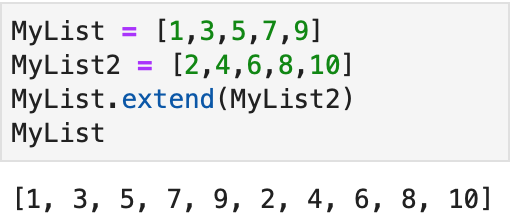To insert elements to the middle of a list, use the  .insert( )   function.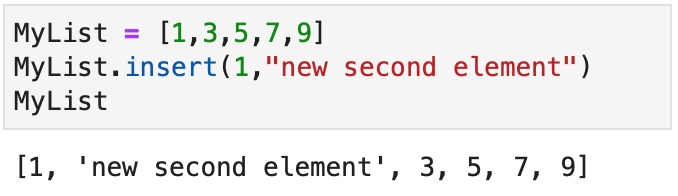The first value in the function is the position to add the element. Remember that the index of a list starts from 0 (i.e., 1 means adding the 2nd element). The second value in the function is the element to be inserted (i.e., the string “new second element”). The element inserted can be of any data type.

To remove elements from a list, use the  .pop( )   function or the .remove( )   function.
The .pop( )   function removes the element by using its index.
The .remove( )   function removes the element by using the value of the element.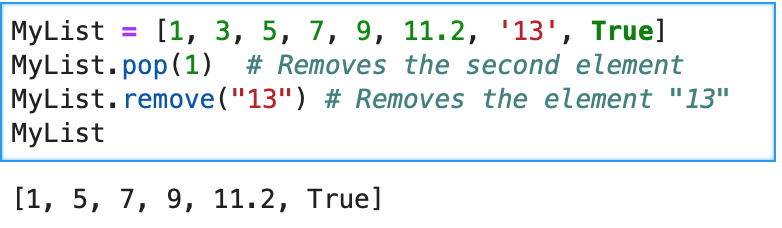### Tuple

tuple     Tuples are ordered lists with immutable elements. The elements of a tuple are put in parentheses.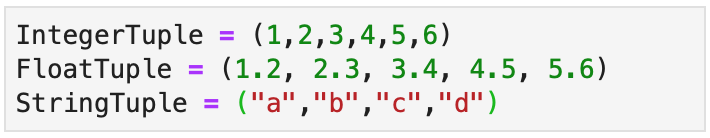Operations of tuples are the same as lists except that elements of tuples are immutable.

Thus, trying to change an element of a tuple results in an error.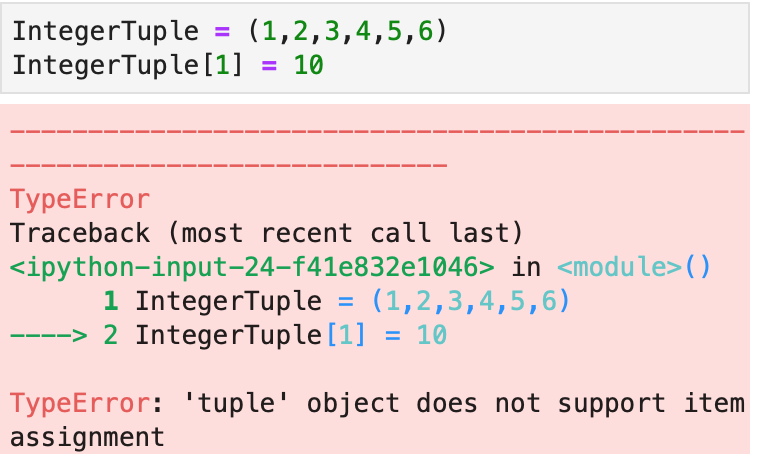### Dictionary

dict     Dictionaries, as suggested by the name, contain pairs of keys and values (such as finding the word “Apple” using the letter “A”). The pairs of keys and values are put inside curly brackets and each key and value are separated by a colon   :   .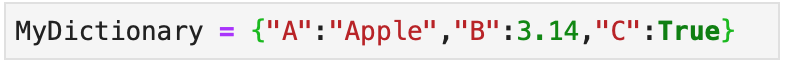To access the elements of a dictionary, use the format DictionaryName[KeyName].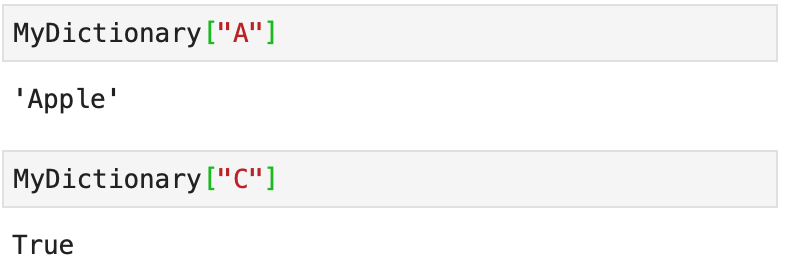Dictionary keys are immutable but the values are mutable.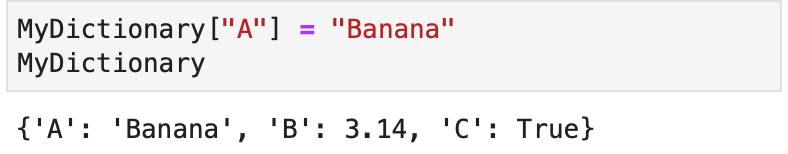To add elements to a dictionary, add the pair of keys and values directly to the dictionary.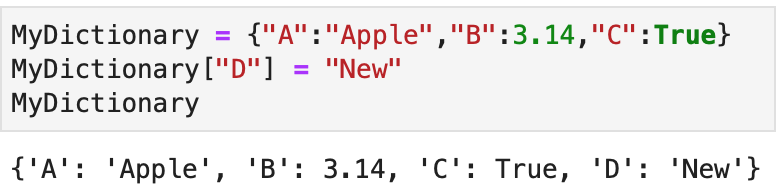## Working with Data

### Checking Data Type

To check the data type of a variable, use the   type( )    function.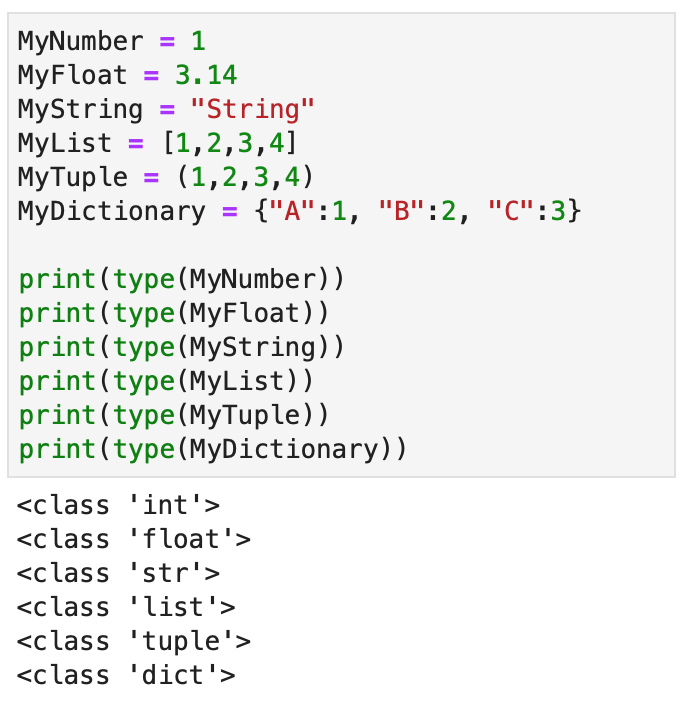To change the data type, use the format   Desired_Data_Type(variable)   .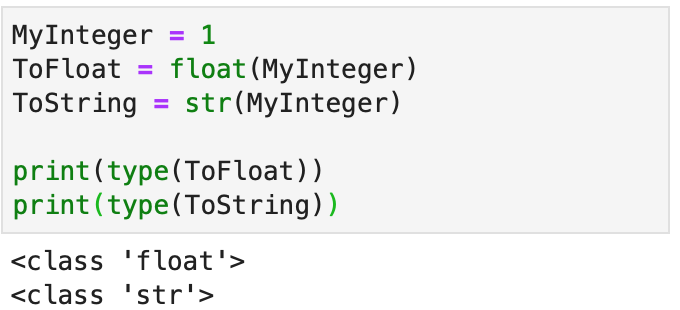Changing a floating-point number to an integer rounds down the number.

A single element cannot be converted to a list, tuple, or dictionary and vice versa. Also, a string with a decimal point (e.g., “6.28”) can only be converted to a floating-point number, not an integer.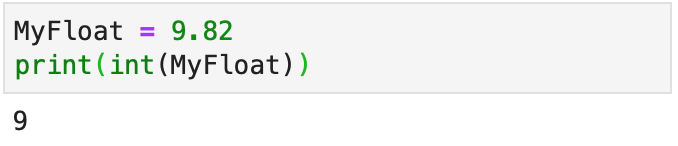Operator Description Example
+ Addition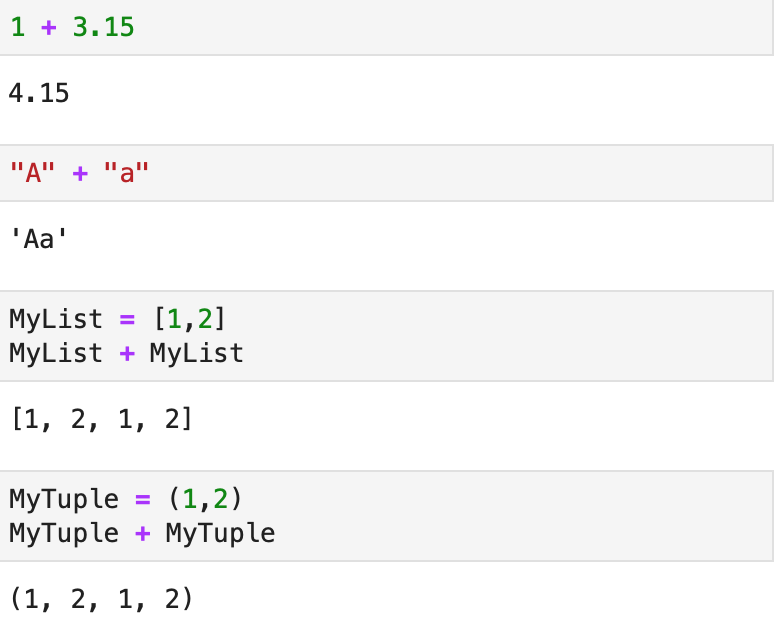Subtraction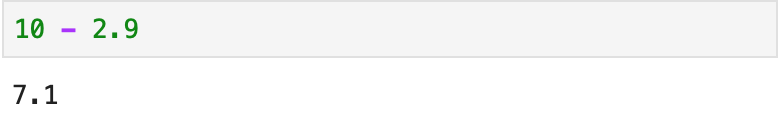* Multiplication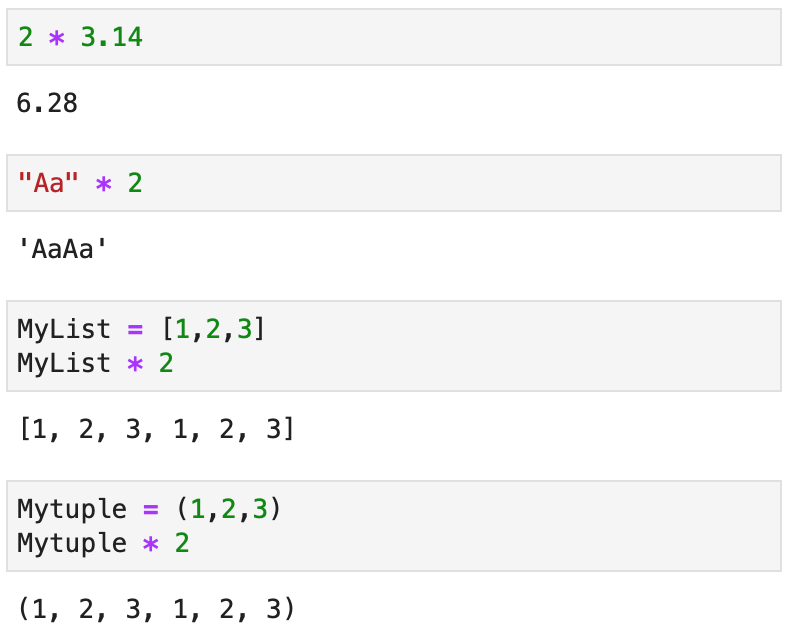/ Division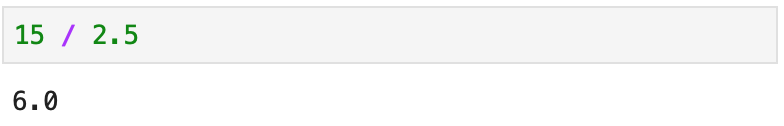** Power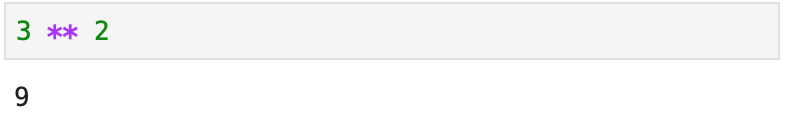% Remainder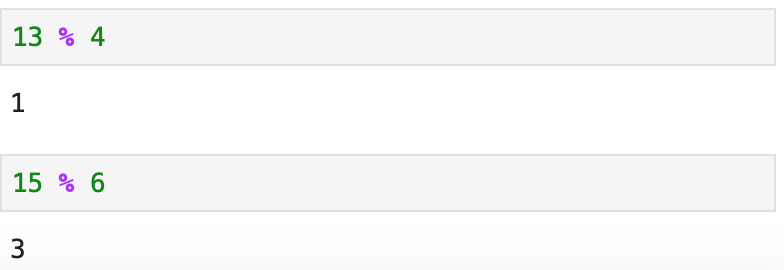// Integral Quotient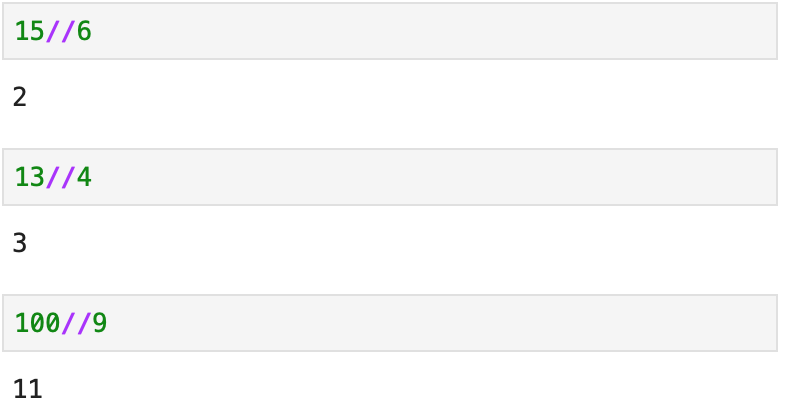If different basic operators are used in the same code cell, they are executed in the following order:

Order of operatiom Basic Operator
1st ( )
2nd **
3rd * / // %
4th + –

Example: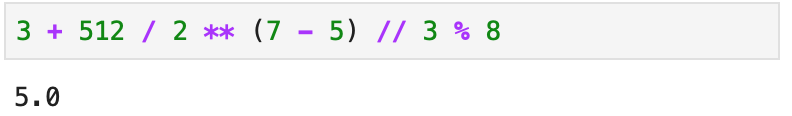The calculation in parentheses   ( )  is performed first to yield 2 (7 – 5). The   **   operator is then performed to yield 4 ( 22 ). The   /   ,   //   and   %   are of the same order and are thus executed from left to right to yield 512 / 4 = 128, 128 // 3 = 42, 42 % 8 = 2. Lastly, the   +   is executed to yield the final result of 5.

## Exercise

Turn the list ListA = [1,3,5,7,9] to a list [1,2,3,4,5,6,7,8,9,10].

Here are three different options:

1. Using   .insert( )   :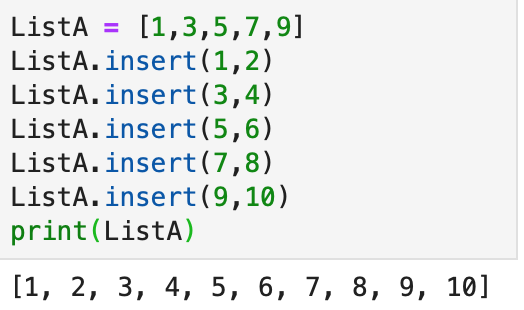2. Using   .extend( )   :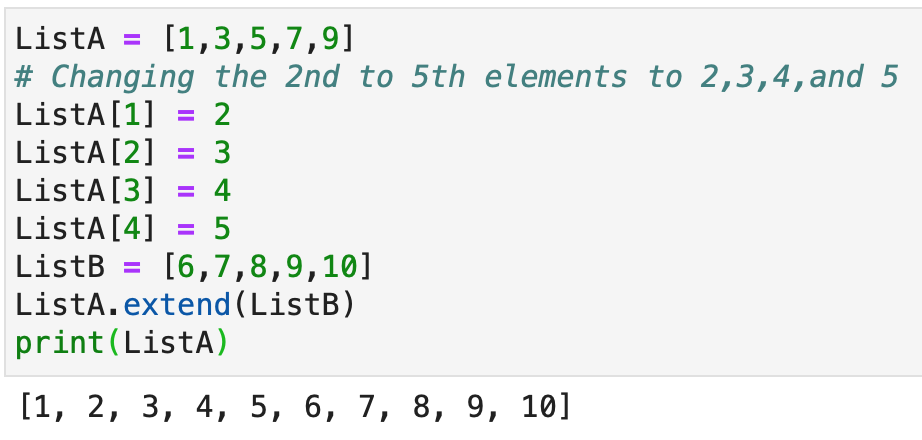3. Using   .append( )   :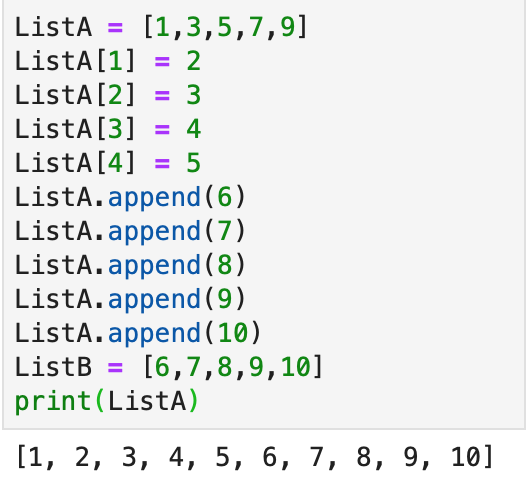## Learning Python Data Analytics with Gleim

At Gleim, we know learning data analytics is vital for future accounting professionals. That is why we are offering these series of data analytics blogs and continually updating all of our CPA, CMA, and CIA Review materials with the necessary information you need to pass your exams.

We’ll continue our weekly blog series. Check back regularly for exam news and study tips!

Python Lessons
Python Basics
Conditional Statements
Loops
Functions and Modules
Numerical Python (NumPy)
Pandas Basics
Pandas Data Capture and Cleansing
Merging and Grouping
Manipulating Text and Datetime Data
Visualization
Web Scraping
Errors and Exceptions